# Second Order Differential Equation Example

Solve the following second order differential equation:

Solution

Let’s write the auxiliary equation: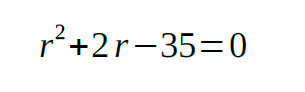Now it can be rewritten in the next form: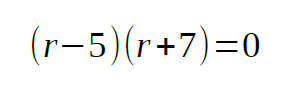So, the roots are: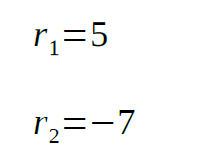The general solution for the differential equation is: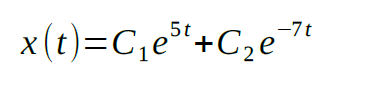Finally, we should find the unknown coefficients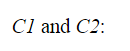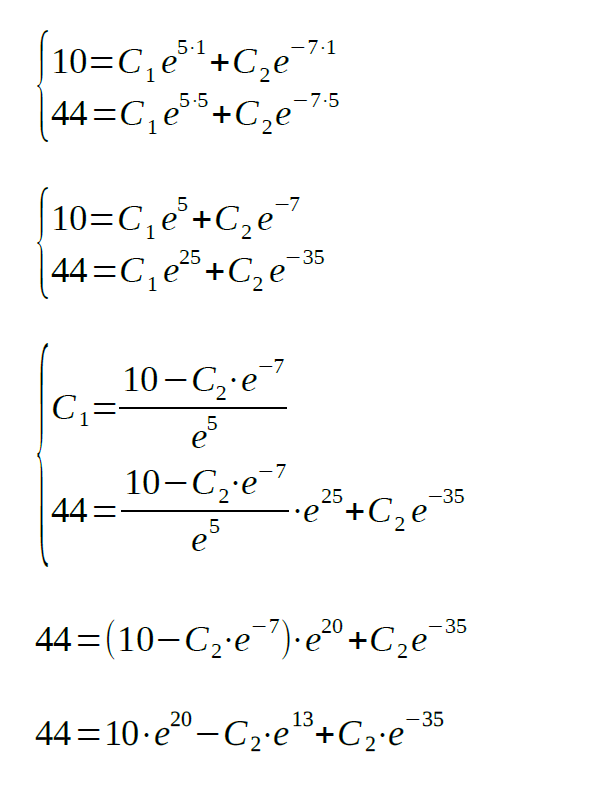2. Solve the following second order differential equation: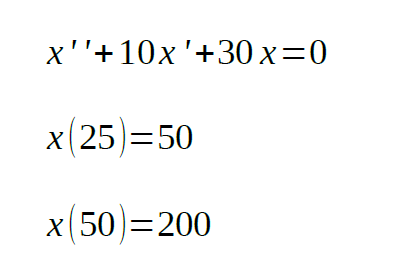Solution

The auxiliary equation will be: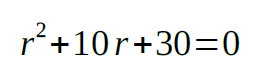The roots of the auxiliary equation will be: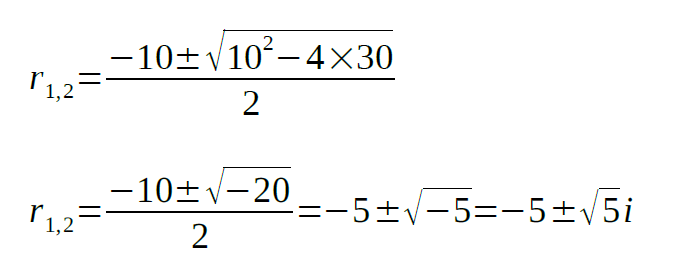Thus, the solution for the differential equation is: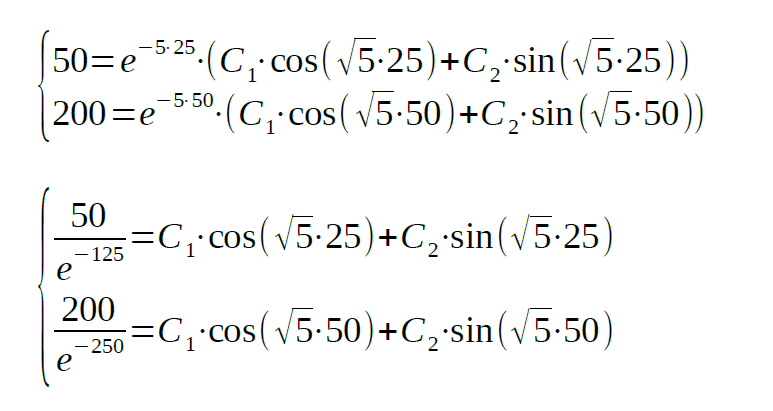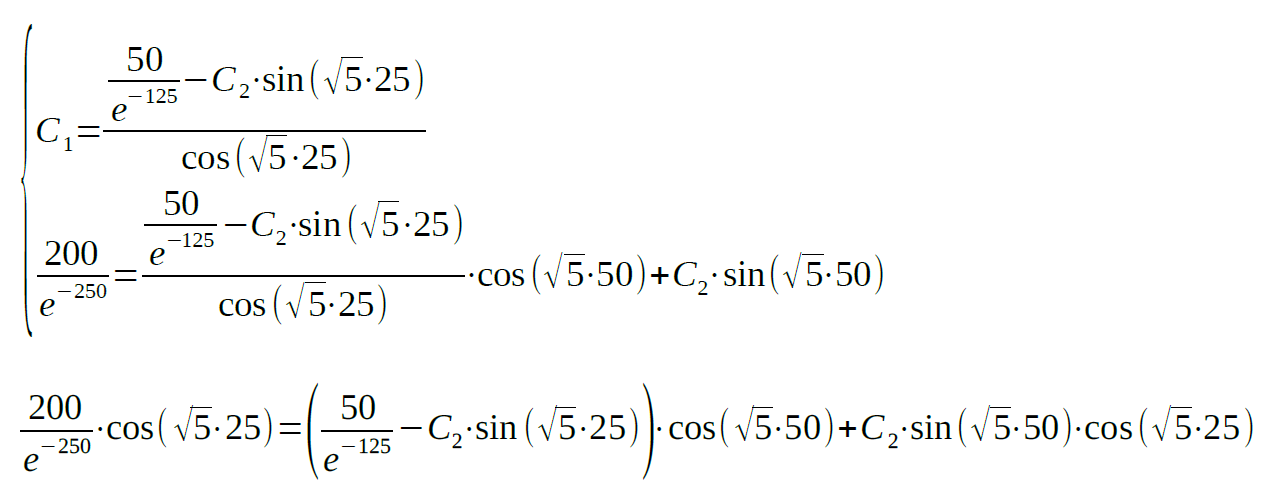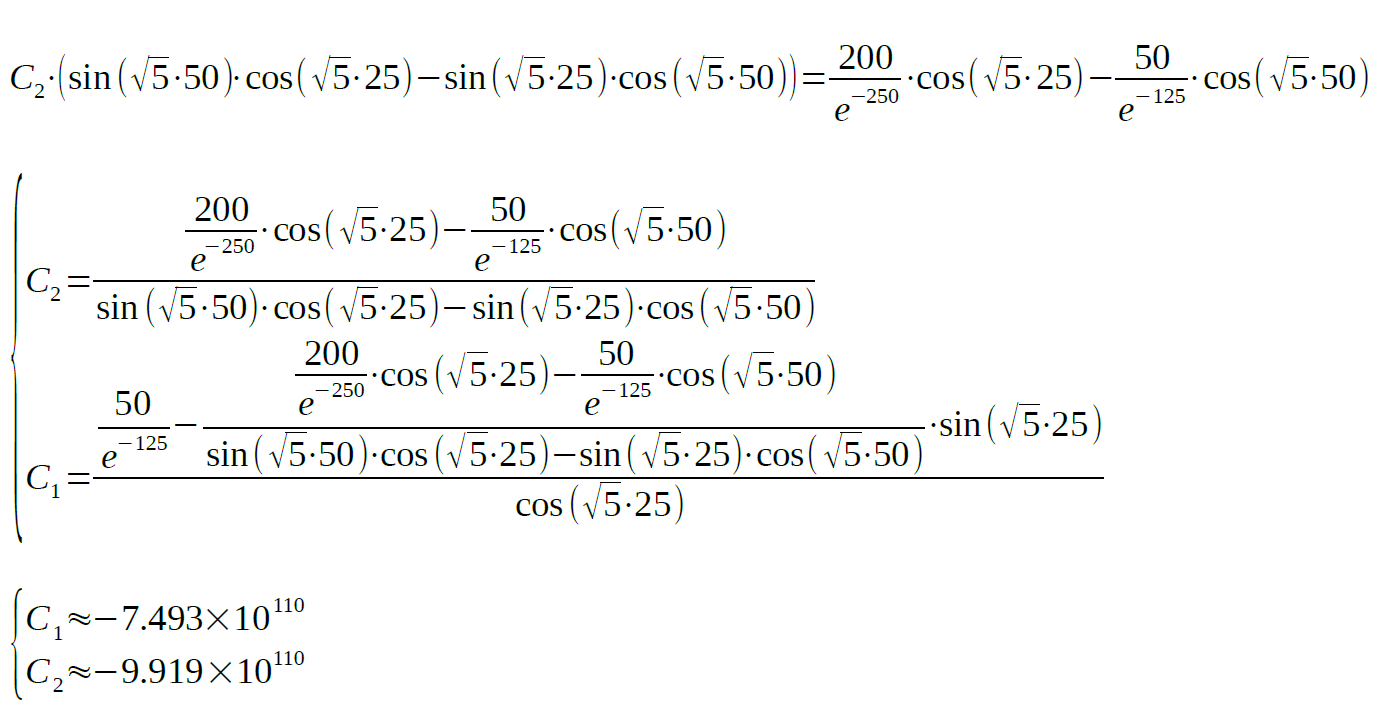Mathematics often becomes a major pain, especially when you have no time to do your homework. And second order differential equations will fill your nightmares, along with the imposing deadlines. That’s not how it’s supposed to be! You have to deal with this mathematical horror once and for all.

Check our solution of second order differential equation provided by a professional technical writer. It should make everything clearer for you. Our team of experts works hard to help as many students as possible. We take orders 24/7 and provide high-quality academic assistance.

If you need math assignment help with your homework or any other discipline, fill in the order form. Assign one of our experts for your order and get better grades!